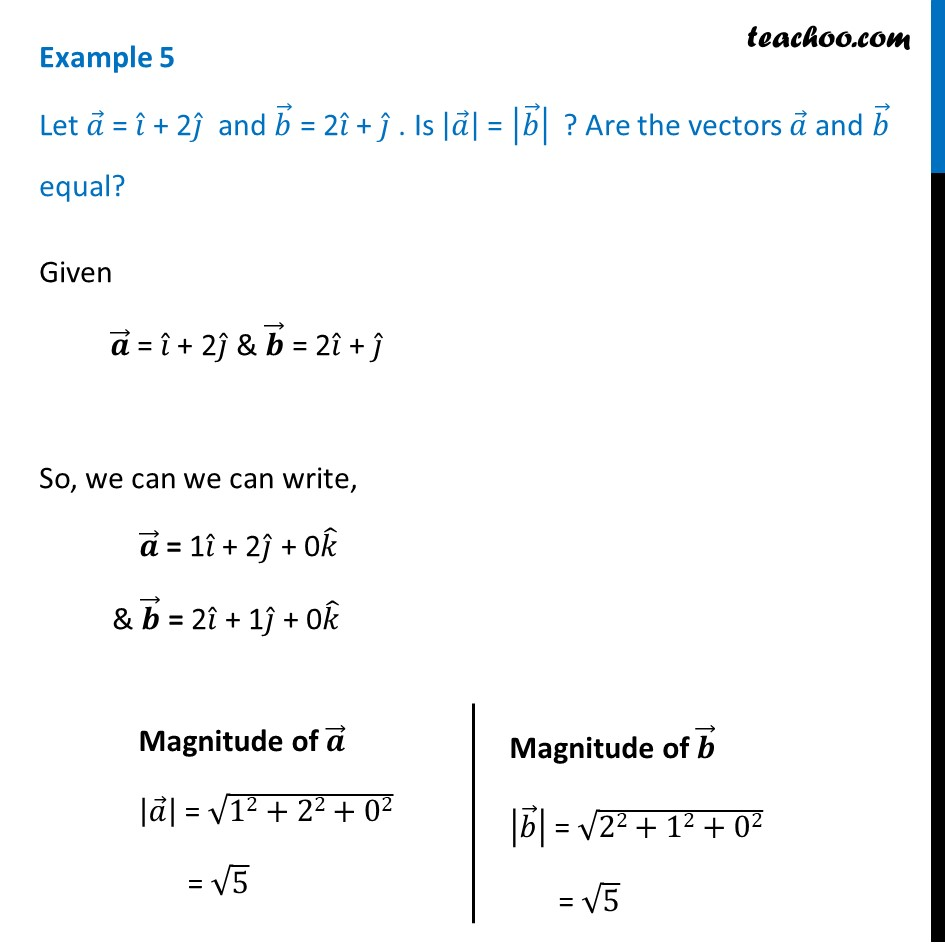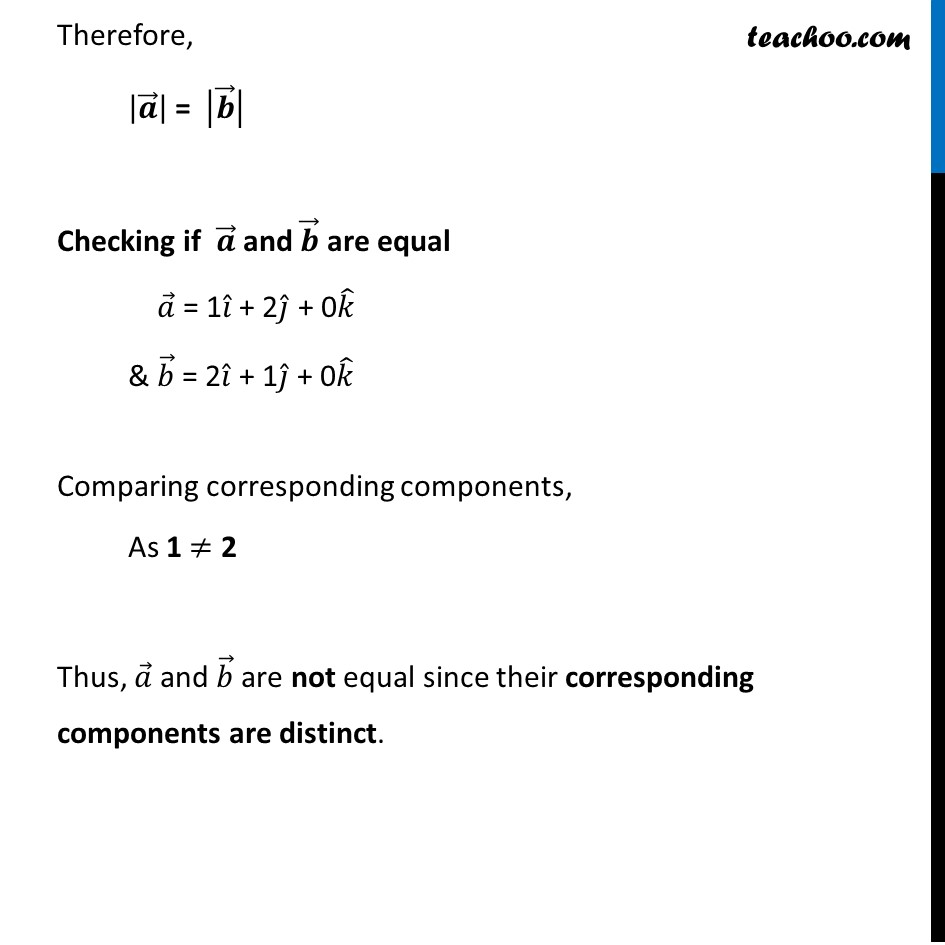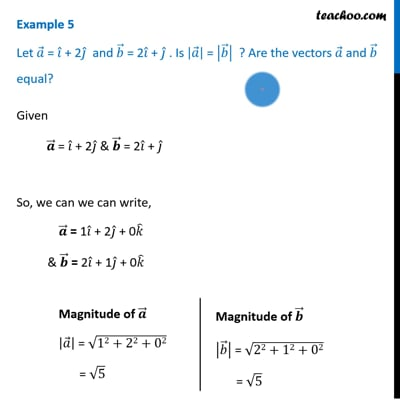Examples

Chapter 10 Class 12 Vector Algebra
Serial order wiseThis video is only available for Teachoo black users

Solve all your doubts with Teachoo Black (new monthly pack available now!)

### Transcript

Example 5 Let 𝑎 ⃗ = 𝑖 ̂ + 2𝑗 ̂ and 𝑏 ⃗ = 2𝑖 ̂ + 𝑗 ̂ . Is |𝑎 ⃗ | = |𝑏 ⃗ | ? Are the vectors 𝑎 ⃗ and 𝑏 ⃗ equal? Given 𝒂 ⃗ = 𝑖 ̂ + 2𝑗 ̂ & 𝒃 ⃗ = 2𝑖 ̂ + 𝑗 ̂ So, we can we can write, 𝒂 ⃗ = 1𝑖 ̂ + 2𝑗 ̂ + 0𝑘 ̂ & 𝒃 ⃗ = 2𝑖 ̂ + 1𝑗 ̂ + 0𝑘 ̂ Magnitude of 𝒂 ⃗ |𝑎 ⃗ | = √(12+22+02) = √5 Magnitude of 𝒃 ⃗ |𝑏 ⃗ | = √(22+12+02) = √5 Therefore, |𝒂 ⃗ | = |𝒃 ⃗ | Checking if 𝒂 ⃗ and 𝒃 ⃗ are equal 𝑎 ⃗ = 1𝑖 ̂ + 2𝑗 ̂ + 0𝑘 ̂ & 𝑏 ⃗ = 2𝑖 ̂ + 1𝑗 ̂ + 0𝑘 ̂ Comparing corresponding components, As 1 ≠ 2 Thus, 𝑎 ⃗ and 𝑏 ⃗ are not equal since their corresponding components are distinct.